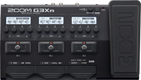# New Order - Blue Monday

Discussion in 'Zoom G3n/G3Xn/G5n' started by Ken Eckert, Nov 25, 2022.

1. New Order - Blue MondayDevice: Zoom G3Xn
Firmware: 2.20

Name on device: New Order
Optimized for: Phones/Speaker

Effects chain:This patch simulates the sharp, bassy tone used on "Blue Monday" by New Order. It will also work on a Zoom G1-Four with modification.

Effect: "MATCH30" (Amp simulator), active - "yes"
"Gain 1" = 40
"Bass 1" = 100
"Treble 1" = 30
"Gain 2" = 5
"Tone 2" = 1
"Cut" = 0
"Volume" = 50
"SOLO" = 3

Effect: "Gt GEQ 7" (Filter), active - "yes"
"100 Hz" = 6.0
"200 Hz" = 0.0
"400 Hz" = 0.0
"800 Hz" = 0.0
"1.6 kHz" = 12.0
"3.2 kHz" = 12.0
"6.4 kHz" = 6.0
"Volume" = 80
"100 Hz" = 0.0
"200 Hz" = 0.0
"400 Hz" = 0.0
"800 Hz" = 0.0
"1.6 kHz" = 0.0
"3.2 kHz" = 0.0
"6.4 kHz" = 0.0
"Volume" = 80
"Parameter set" = A

Effect: "Phaser" (Modulation), active - "yes"
"Color" = 4 STG
"Depth" = 20
"Rate" = 12
"Resonance" = 50

Effect: "AnalogDly" (Delay), active - "yes"
"Time" = 70
"F.B." = 15
"Mix" = 50
"Tail" = On

Effect: "Plate" (Reverb), active - "yes"
"PreD" = 17
"Decay" = 25
"Mix" = 40
"Tail" = On

Patch Volume: 100